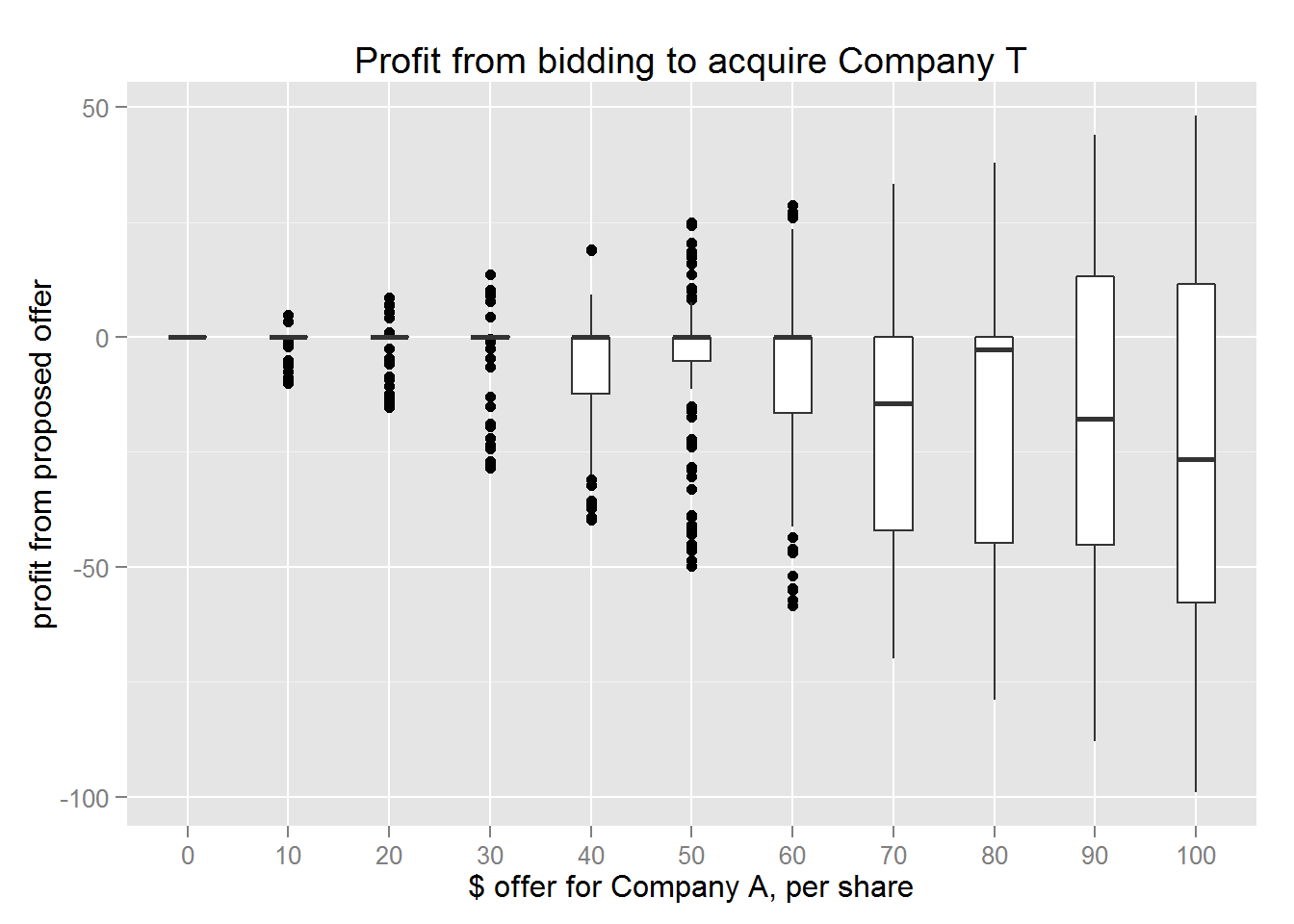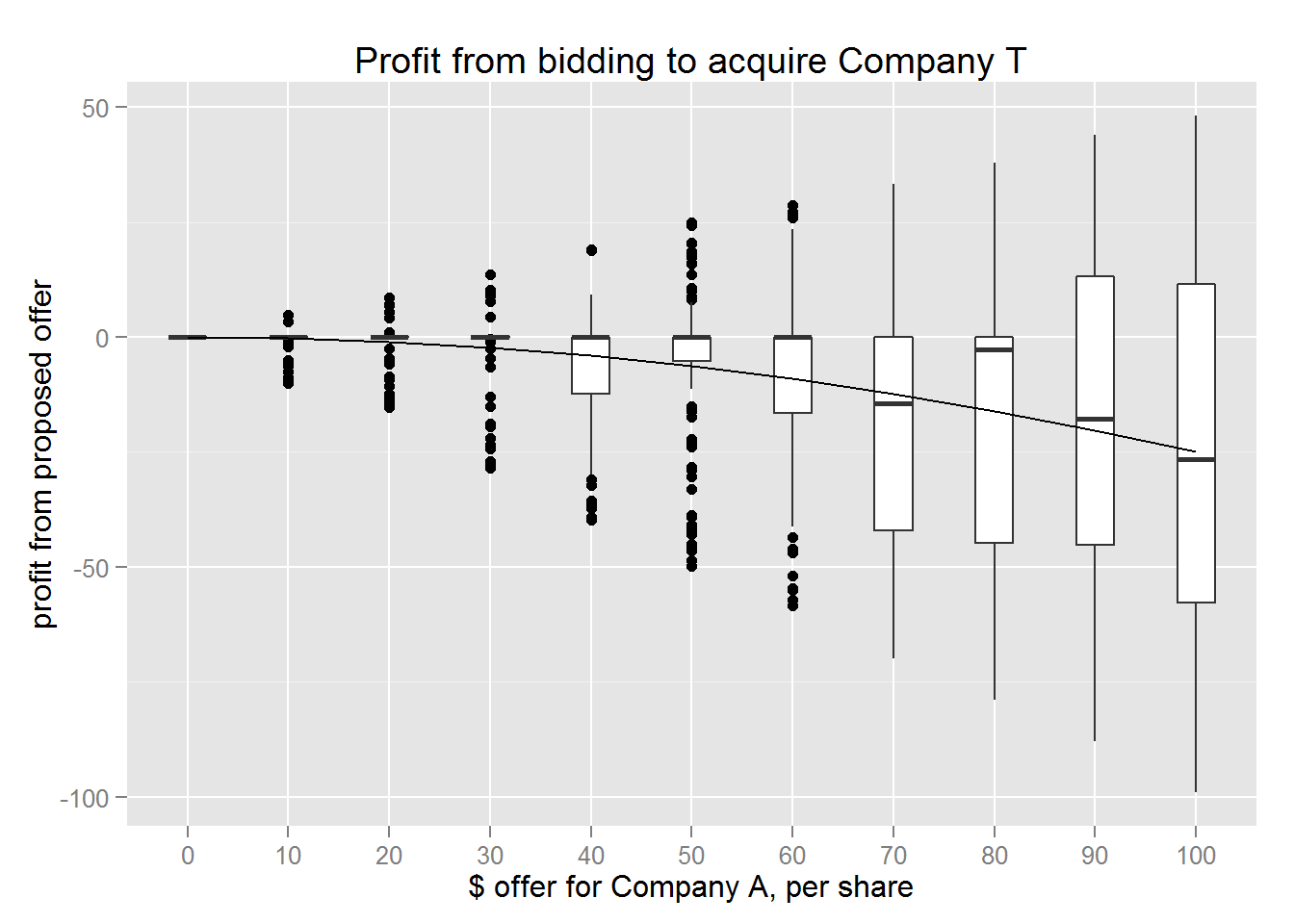29 May 2015

# Negotiation Genius

A chapter from Deepak Malhotra and Max H. Bazerman’s Negotiation Genius provides the following challenge:

Company T is about to make a discovery that will result in their stock price being a random number between $0 and$100.

As CEO of Company A, you know that purchasing Company T will be worth, due to synergies, 150% of its stock price.

(If the Company T is $80/share, it is worth$120/share to you)

You would like to make an offer to acquire Company T.

The trick is that you have to make your bid before the discovery takes place, but Company T will decide to accept your offer or not after the discovery.

How much should you bid to take over Company T?

According to the book, the vast majority of MBA students get this question wrong.

I thought it was an interesting statistical problem, and analyze both mathematically, and via a simulation.

## Simulation

# Simulating the challenge from Negotiation Genius
library(dplyr)
set.seed(5000) #suite
bidToAcquireCompany <- function(n_sim = 30, min_val = 0, max_val = 100,offer_val = 60,valueAdd=1.5) {
# input:
#     n_sim: number of simulations to run
#     min_val: minimum possible outcome of surprise
#     max_val: maximum possible outcome of surprise
#     offer_val: the (currently scalar) offer to present the company
#     valueAdd: scalar of multiple the acquiring company will make off of the synergies.
outcomes = runif(n_sim, min=min_val,max=max_val)
offer = rep(offer_val,n_sim)
result = offer > outcomes
profit = result * ((valueAdd* outcomes) - offer)
return(as.data.frame(cbind(outcomes,offer,result,profit)))
}

res <- bidToAcquireCompany(n_sim=5)
simulation_results <-
seq(from=0,to=100,by=10) %>%
lapply(.,FUN=function(x) {bidToAcquireCompany(offer_val=x,n_sim=100)}) %>%
do.call(rbind,.)

simulation_summary  <-
simulation_results %>%
group_by(offer) %>%
summarise(mean_profit = mean(profit))

library(ggplot2)

p <- ggplot(data=simulation_results,aes(x=factor(offer),y=profit)) +
xlab("\$ offer for Company A, per share") +
ylab("profit from proposed offer") +
ggtitle("Profit from bidding to acquire Company T")

p + geom_boxplot(width=.5)## Math Solution

I’ll use the default parameter values here, can be easily be abstracted

$$T \sim U(0,100)$$

We note that

$$P = 1.5*T - offer$$
if $$T < offer$$

and

$$P = 0$$ if $$T > offer$$

Calculate expected value:

$$E(P) = 1.5* E(T | T < offer) * P(T < offer) + 0 * P(T > offer)$$

$$E(P) = 1.5* E(T') * P(T<offer)$$

where $$T' \sim U(0,offer)$$

$$E(P) = ( \frac{3}{2} * \frac{offer}{2} - offer) * \frac{offer}{100}$$

$$E(P) = ( \frac{3}{4} - 1 ) * \frac{offer^2}{100}$$

$$E(P) = - \frac{offer^2}{400}$$

We’ll calculate this line and draw it in.

math_solution = function(offer) return(- offer^2 / 400)

math_results <- math_solution(seq(from=0,to=100,by=10)) %>% data.frame(profit = .)

p + geom_boxplot(width=.5) +
geom_line(data=math_results,aes(x = 1:11,y=profit))If I have more time, I’ll figure out $$Var(P(offer))$$ as well!